Constructing a triangle using SSS congruency criteria

Practical Geometry
Serial order wise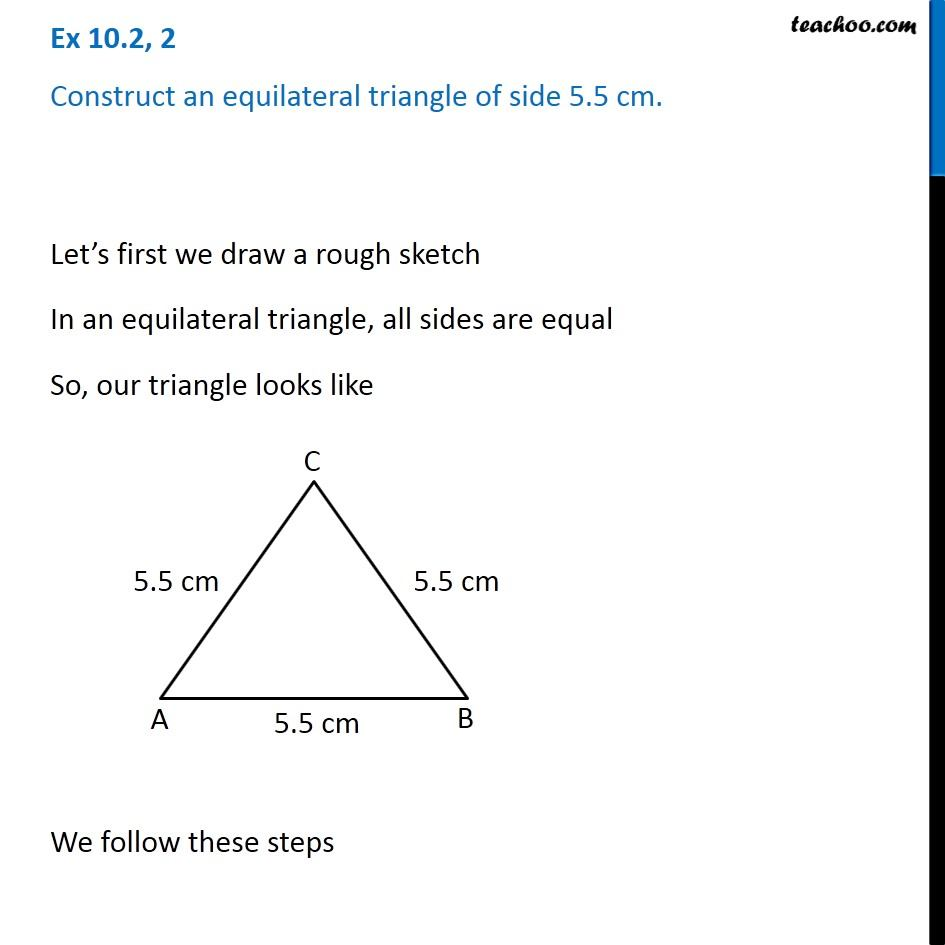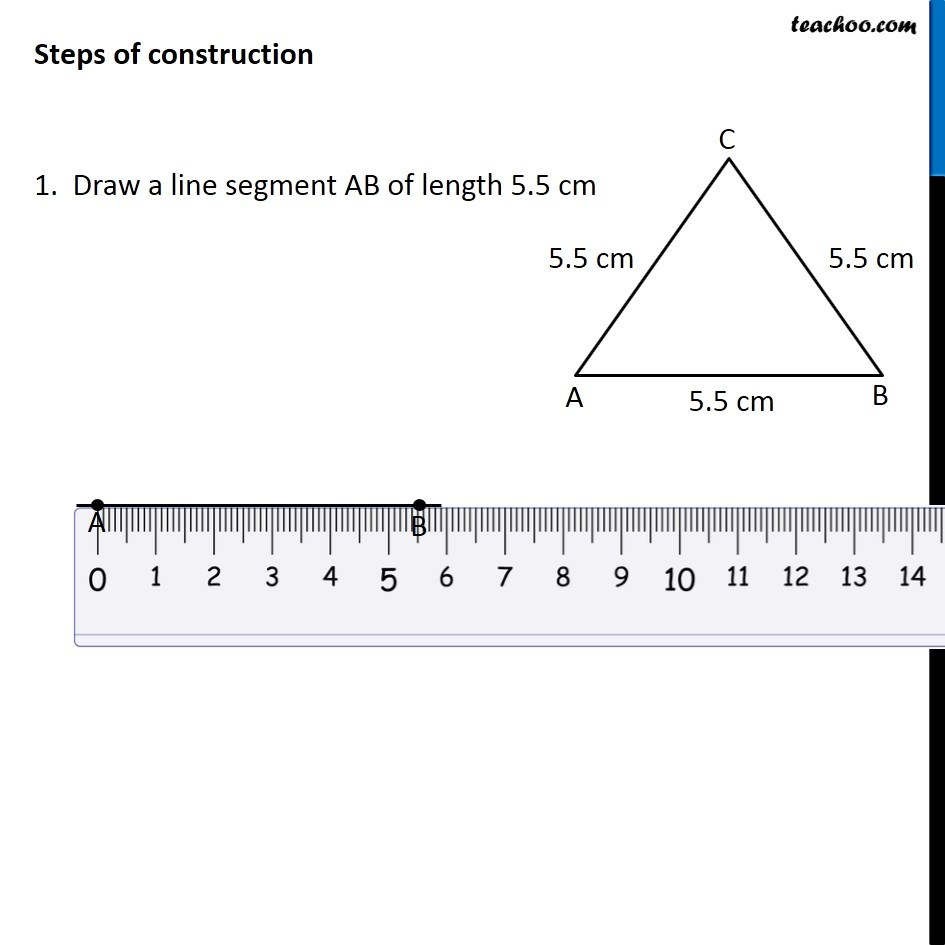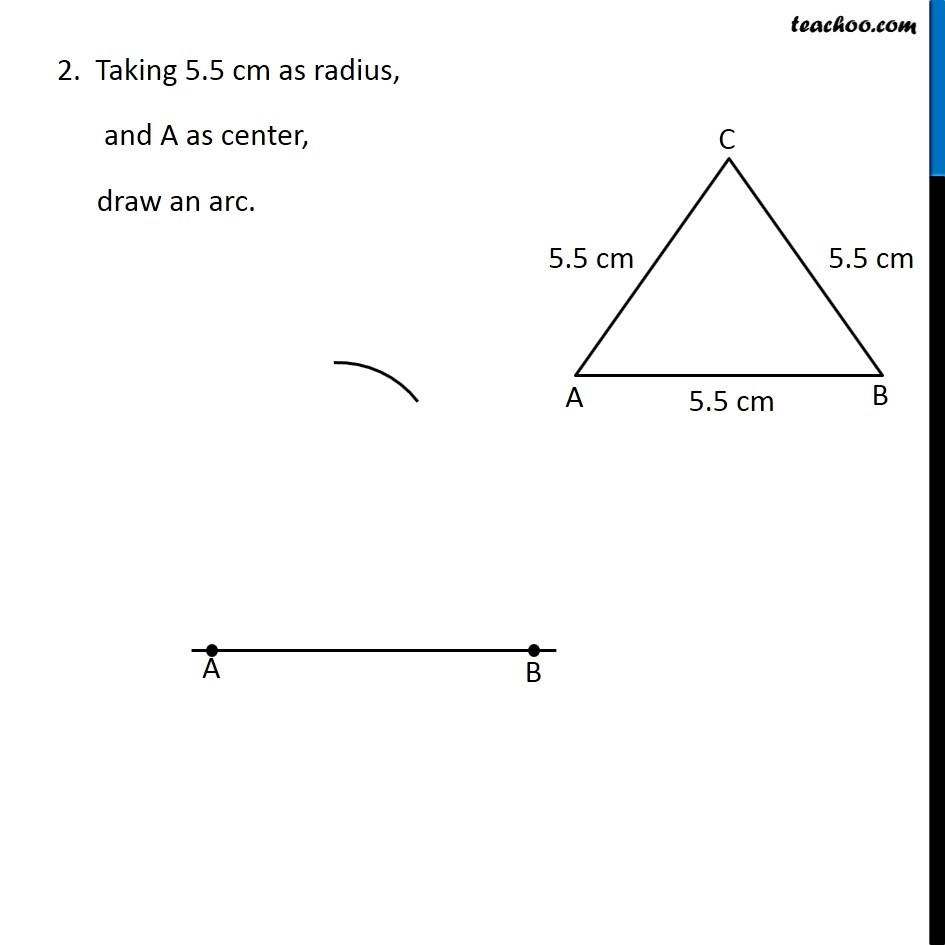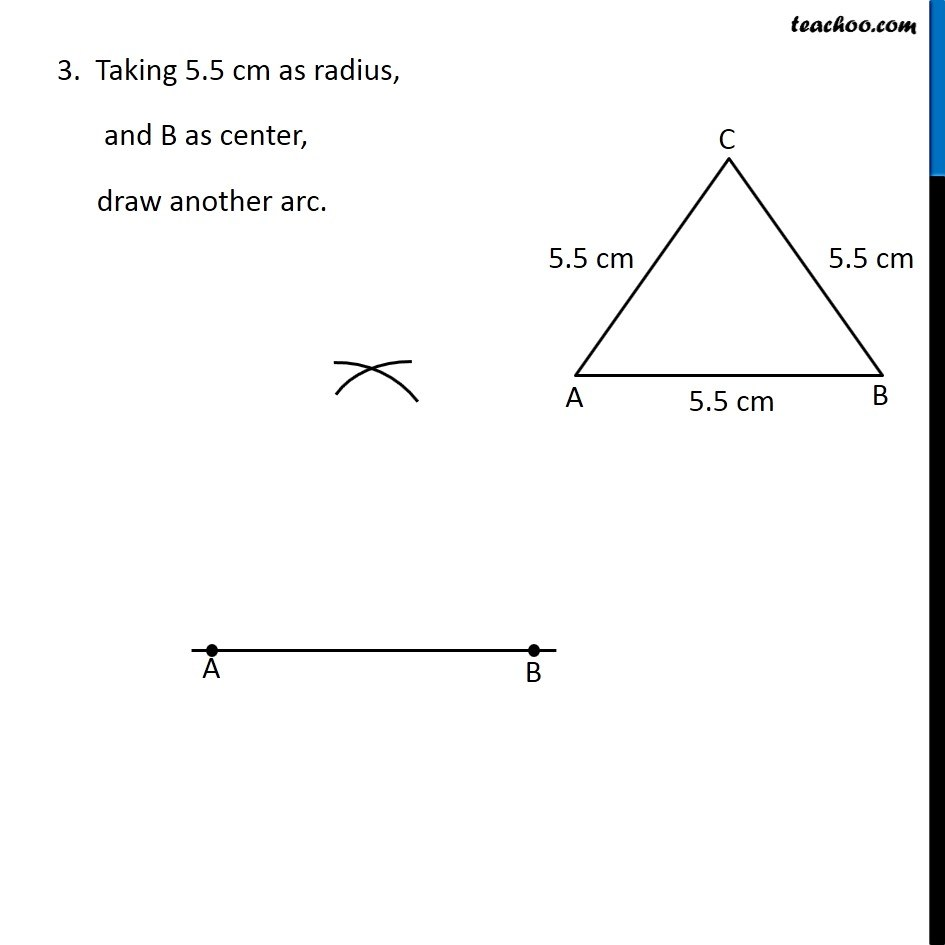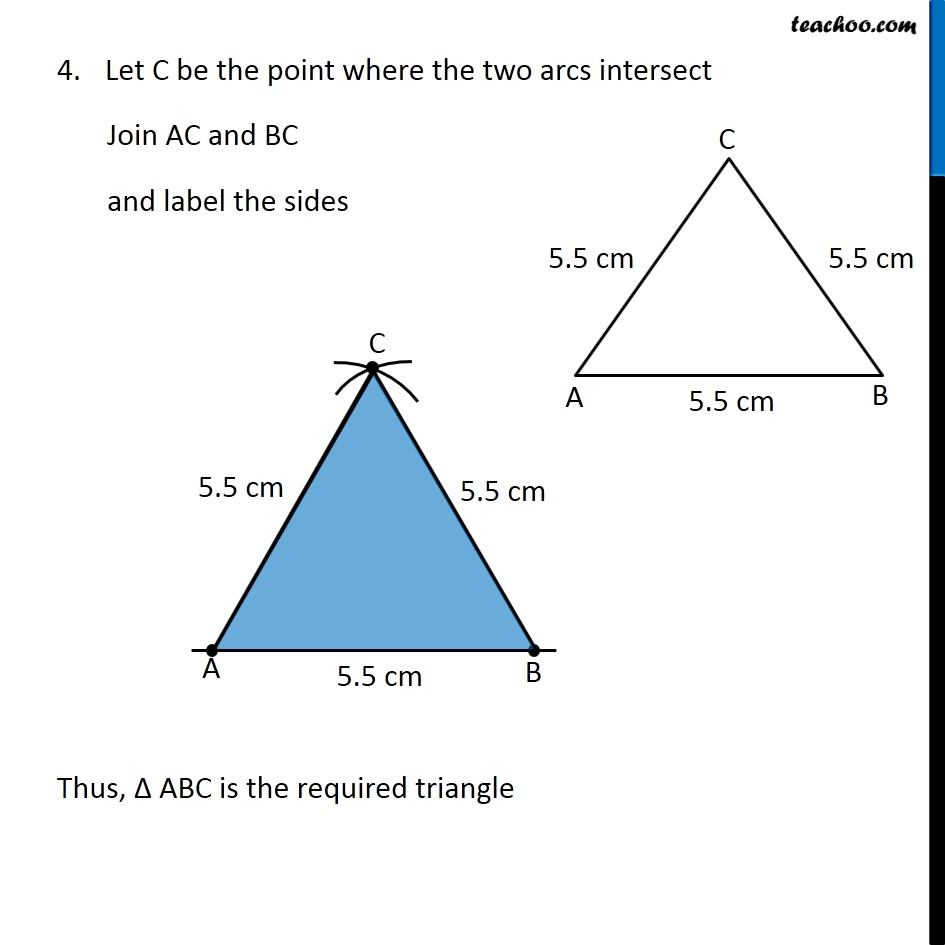Learn in your speed, with individual attention - Teachoo Maths 1-on-1 Class

### Transcript

Question 2 Construct an equilateral triangle of side 5.5 cm. Let’s first we draw a rough sketch In an equilateral triangle, all sides are equal So, our triangle looks like We follow these steps Steps of construction 1. Draw a line segment AB of length 5.5 cm 2. Taking 5.5 cm as radius, and A as center, draw an arc. 3. Taking 5.5 cm as radius, and B as center, draw another arc. Let C be the point where the two arcs intersect Join AC and BC and label the sides Thus, Δ ABC is the required triangle